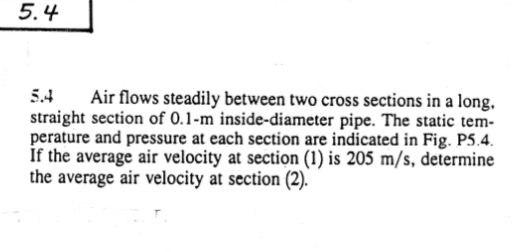# Question Urgent 5.4 5.4 Air flows steadily between two cross sections in a long, straight section of 0.1-m inside-diameter pipe. The static tem- perature and pressure at each section are indicated in Fig. P5.4. If the average air velocity at section (1) is 205 m/s, determine the average air velocity at section (2).QCRNGW The Asker · Electrical Engineering

UrgentTranscribed Image Text: 5.4 5.4 Air flows steadily between two cross sections in a long, straight section of 0.1-m inside-diameter pipe. The static tem- perature and pressure at each section are indicated in Fig. P5.4. If the average air velocity at section (1) is 205 m/s, determine the average air velocity at section (2).
More
Transcribed Image Text: 5.4 5.4 Air flows steadily between two cross sections in a long, straight section of 0.1-m inside-diameter pipe. The static tem- perature and pressure at each section are indicated in Fig. P5.4. If the average air velocity at section (1) is 205 m/s, determine the average air velocity at section (2).